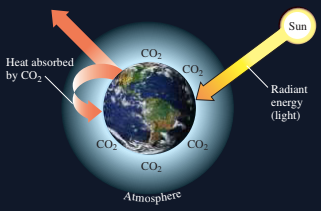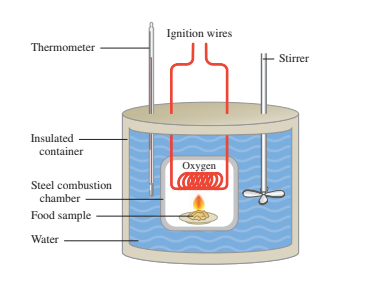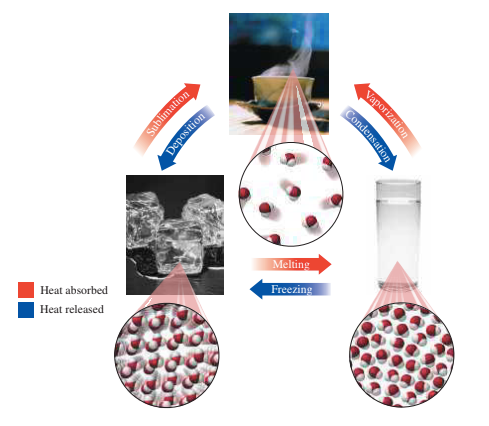# 3.1 - Classification of Matter

• Matter is anything with weight and space.

• Matter shall be classed as a mixture or pure substance.

• Fixed compositions have pure substances or compounds and variable compounds have mixtures.

• The substances can be separated by physical methods in mixtures.# 3.2 - States and Properties of Matter

• Solid, liquid, and gas are the three states of matter.

• A physical feature is a feature of a substance because it can be observed or measured without affecting its identity.

• When physical properties change, the composition of the substance does not change. Physical changes happen.

• A chemical property shows a substance's ability to switch to another.

• When a substance with new physical and chemical properties is formed, a chemical change takes place.

# 3.3 - Temperature

• The temperature in science is measured in Celsius (°C) or kelvins (K).

• On the Celsius scale, the point of freeze (0 °C) and the point of boiling (100 °C) are 100-unit water.

• There are 180 units in scale Fahrenheit between the frostbite (32 °F) and the hotbed (212 °F) of the water.

• The temperature of Fahrenheit by the equation TF = 1.8(TC) + 32 is related to the Celsius temperature.

• The SI unit, kelvin, is associated by equation TK = TC + 273 with the Celsius temperature.

# 3.4 - Energy

• The ability to work is energy.

• Potential energy is energy stored; kinetic energy is moving energy.

• Calorie (cal), kilocalorie (kcal), joule (J), and kilojoule are common energy units (kJ).

• One calorie is 4.184 J.# 3.5 - Energy and Nutrition

• The calorie in nutrition is equal to 1 kcal or 1000 calories of energy.

• The energy of a food is the sum of carbohydrates, fat, and protein kilocalories or kilojoules.# 3.6 - Specific Heat

• Specific heat is the amount of power needed to exactly 1 g of a substance to increase its temperature by 1 °C.

• Through the multiplication of the mass, temperature change, and specific warmth the heat is lost or recovered by a substance.

# 3.7 - Changes of State

• A fusion occurs when the solid particles absorb sufficient energy to break down and form a liquid.

• Fusion thermal means the amount of energy necessary to precisely convert 1 g of solid into liquid.

• For water, it is necessary to melt 1 g of ice or to freeze 1 g of water, cal (334 J).

• Evaporation occurs when particles absorb sufficient energy in a fluid state to separate and form gas particles.

• Boiling at the boiling point is the vaporization of the liquid.

• The amount of heat required to convert 1 g of fluid to vapor is the heat of the vaporization.

• Sublimation is a process through which solid changes into a gas directly.

• A heating or cooling curve shows temperature and condition changes when heat is added to or removed from a material.

• Changes to the state are shown in plateaus on the graph.

• The sum of the energy calculations for state change and/or temperature change(s) is the total heat absorbed or removed from a substance undergoing changes in temperature and state.